#Function Repository Resource:

# StringToBoxes

Convert a string into boxes as if it were typed into a notebook

Contributed by: Richard Hennigan (Wolfram Research)
 ResourceFunction["StringToBoxes"][string] converts string into boxes as if it were typed into a notebook.

## Details and Options

ResourceFunction["StringToBoxes"] generates boxes that are similar to what you would get as if typing into a cell that uses BoxData.
You can see how box structures generated by ResourceFunction["StringToBoxes"] would be displayed by using DisplayForm.
Use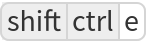(Windows/Linux) or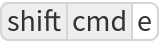(Mac) to view the underlying boxes of a selected cell. »

## Examples

### Basic Examples

Convert a string into boxes:

 In:=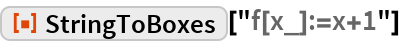Out=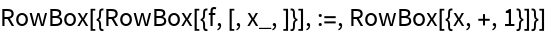View the DisplayForm:

 In:=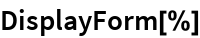Out=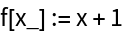View as an input cell:

 In:=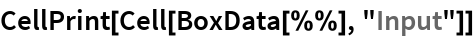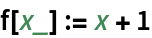The string does not need to be valid syntax:

 In:=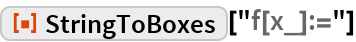Out=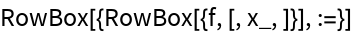In:=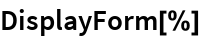Out=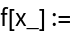### Scope

Convert a linear syntax string into boxes:

 In:=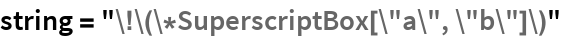Out=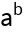In:=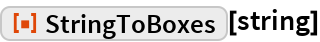Out=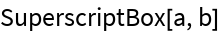In:=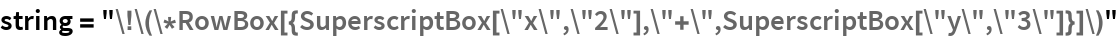Out=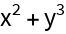In:=Out=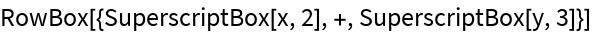The boxes are interpreted as if the string were typed into a notebook:

 In:=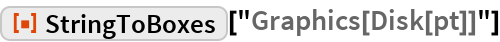Out=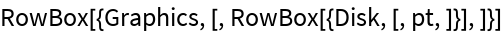In:=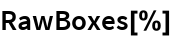Out=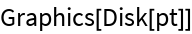Compare to using ToExpression and MakeBoxes:

 In:=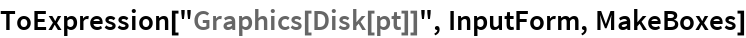Out=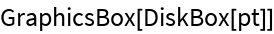This results in an error:

 In:=Out=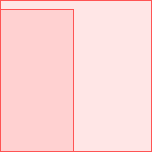StringToBoxes can handle invalid syntax:

 In:=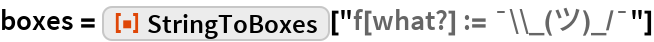Out=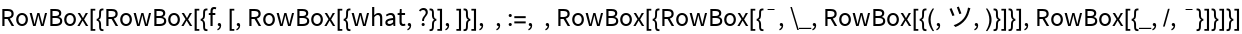This means the resulting boxes will not be valid syntax either:

 In:=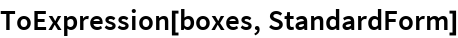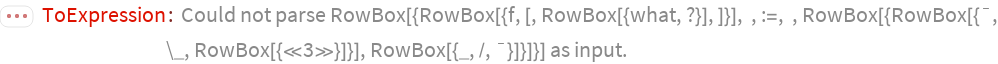Out=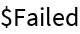The boxes are valid, however, and can be displayed by the front end:

 In:=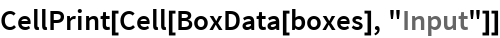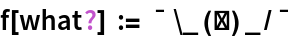### Options

#### PreserveWhitespace

By default, whitespace will be preserved in the output:

 In:=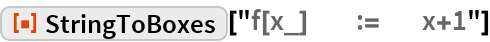Out=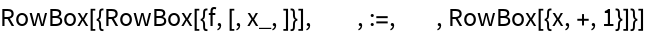In:=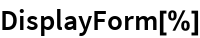Out=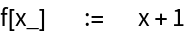Ignore whitespace:

 In:=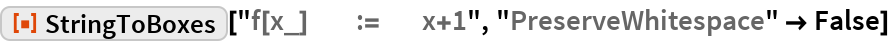Out=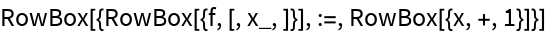In:=Out=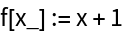## Requirements

Wolfram Language 11.3 (March 2018) or above